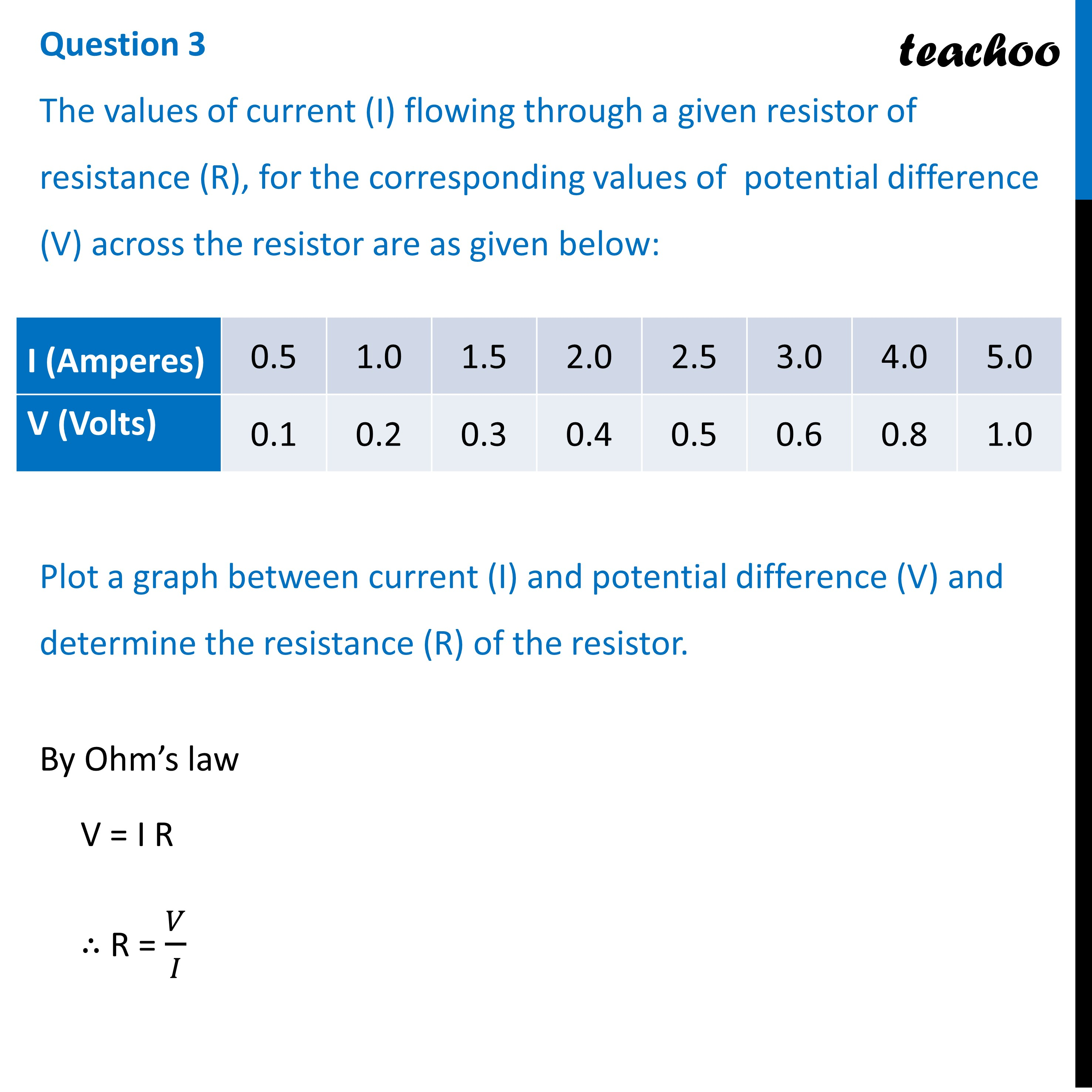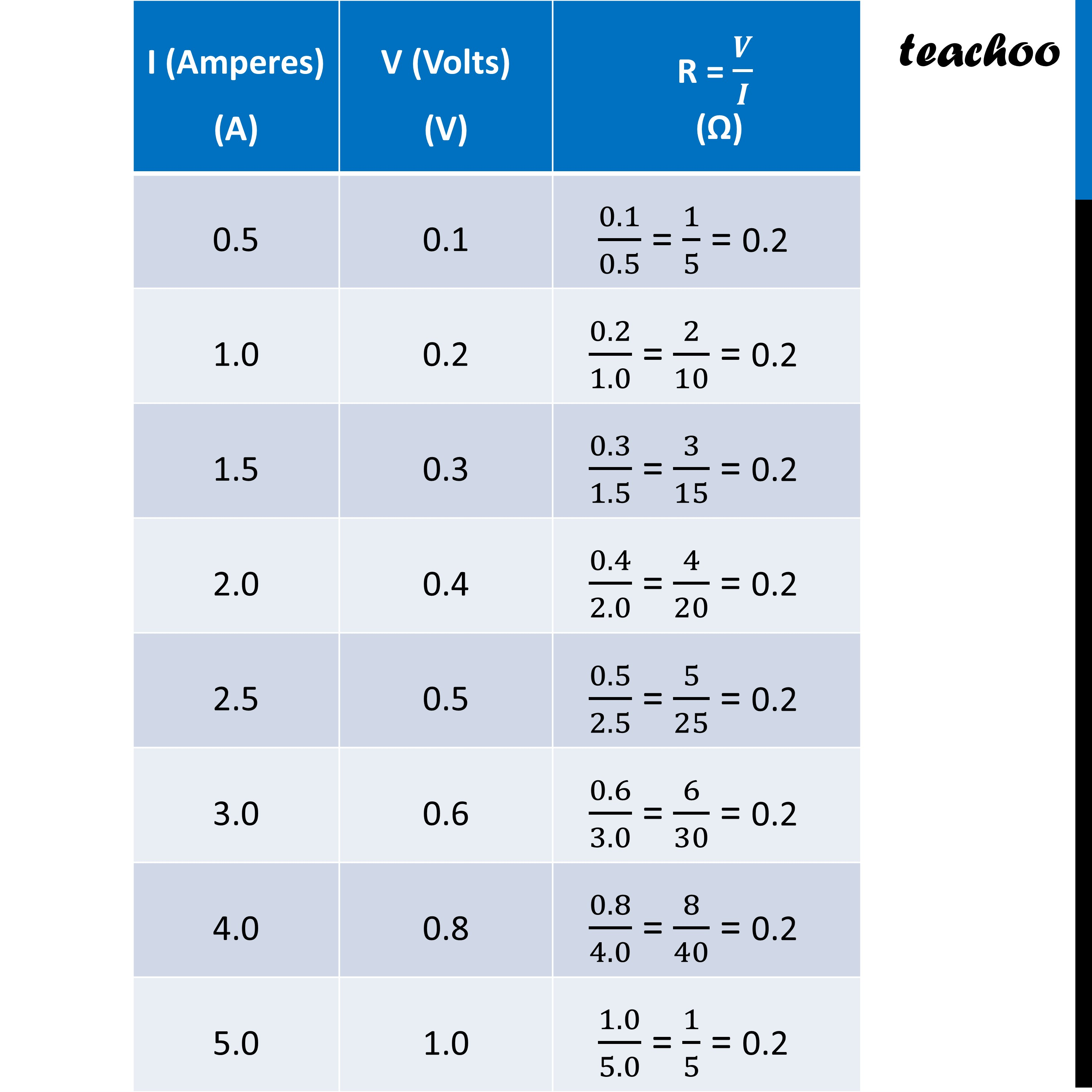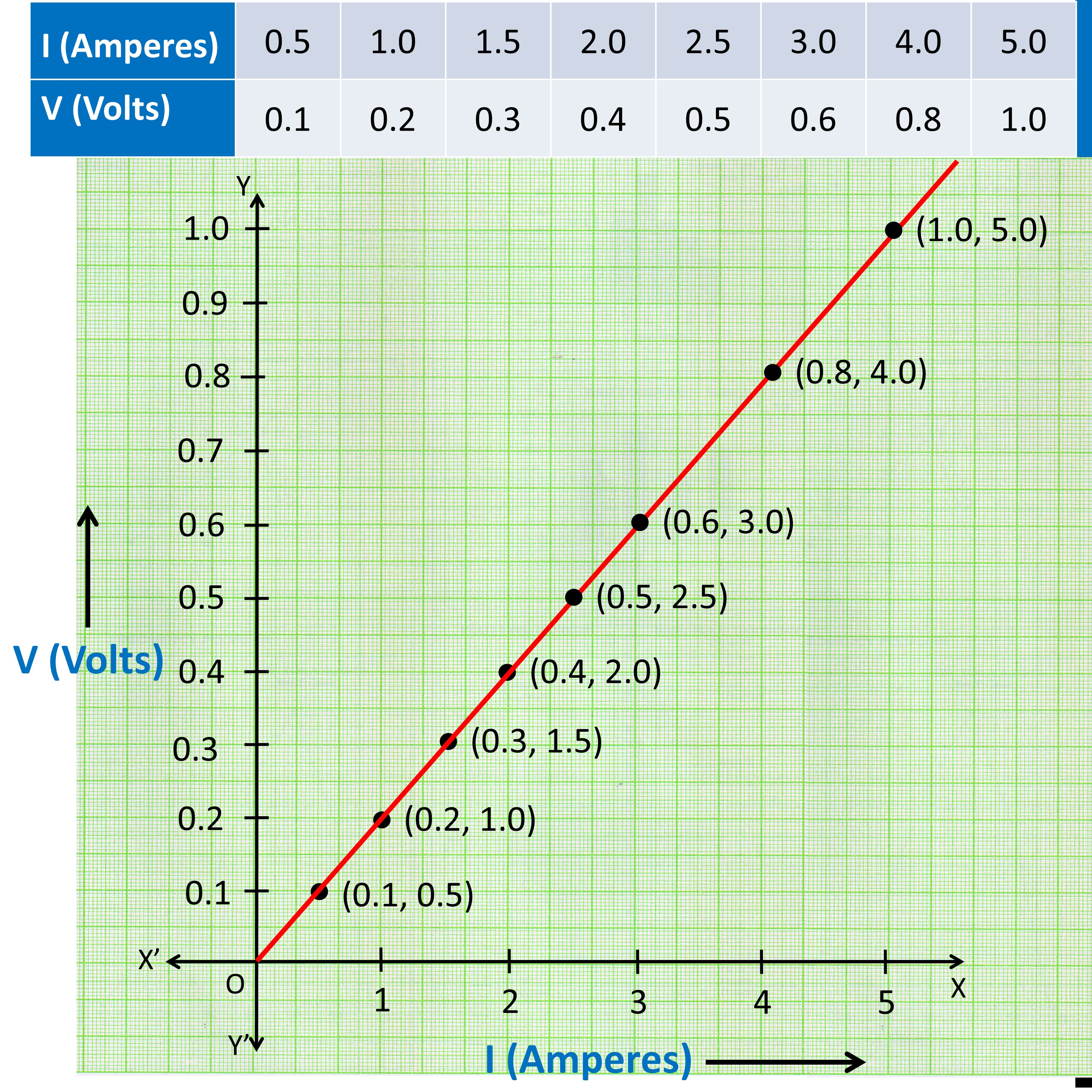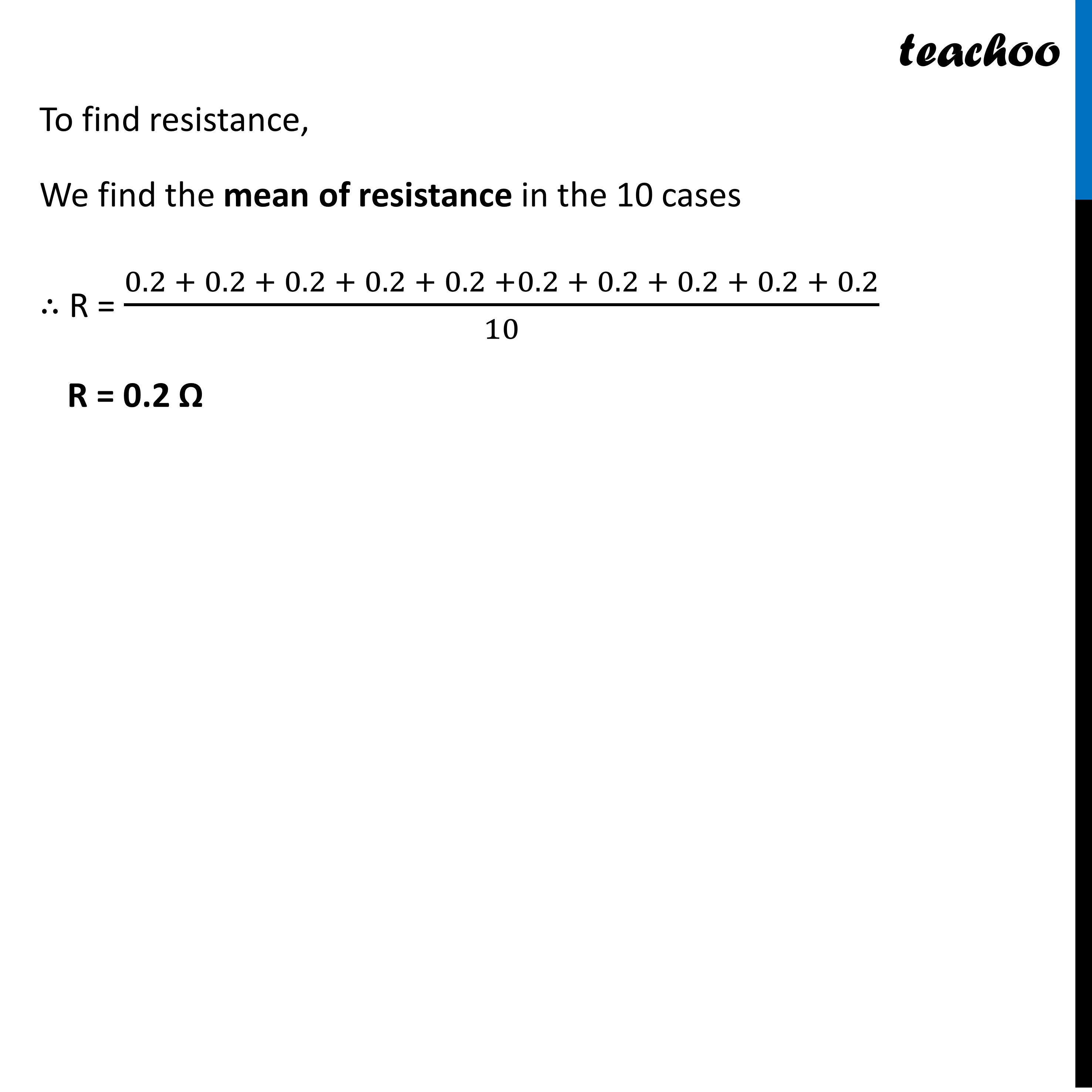Past Year - 2 Mark Questions

Class 10
Chapter 12 Class 10 - Electricity

## The values of current (I) flowing through a given resistor of resistance (R), for the corresponding values of potential difference (V) across the resistor are as given below:

 V(volts) 0.5 1 1.5 2 2.5 3 4 5 I(amepere) 0.1 0.2 0.3 0.4 0.5 0.6 0.8 1

## Plot a graph between current (I) and potential difference (V) and determine the resistance (R) of the resistor.Learn in your speed, with individual attention - Teachoo Maths 1-on-1 Class

### Transcript

Question 3 The values of current (I) flowing through a given resistor of resistance (R), for the corresponding values of potential difference (V) across the resistor are as given below: Plot a graph between current (I) and potential difference (V) and determine the resistance (R) of the resistor. By Ohm’s law V = I R ∴ R = 𝑉/𝐼 V (Volts) I (Amperes) To find resistance, We find the mean of resistance in the 10 cases ∴ R = (0.2 + 0.2 + 0.2 + 0.2 + 0.2 +0.2 + 0.2 + 0.2 + 0.2 + 0.2)/10 R = 0.2 Ω